# Rounding to Significant Figures

Rounding to Significant Figures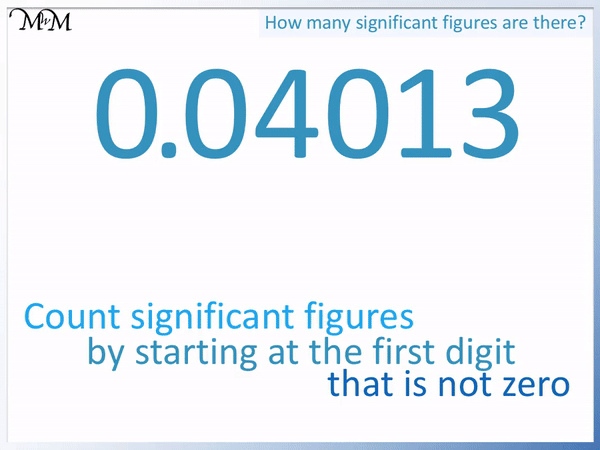• To round the decimal number 0.04013 to 3 significant figures we first need to count the number of significant figures that it has.
• We start counting significant figures from the first number that is not zero.
• The first two digits of 0.04013 are zeros, so we ignore them.
• The first significant digit is 4 because it is the first digit that is not zero.
• So 4 is the first significant figure, 0 is the 2nd, 1 is the 3rd and 3 is the 4th.
• We have 4 significant figures.
• Notice that we count the zero after the 4 because we have started counting at 4.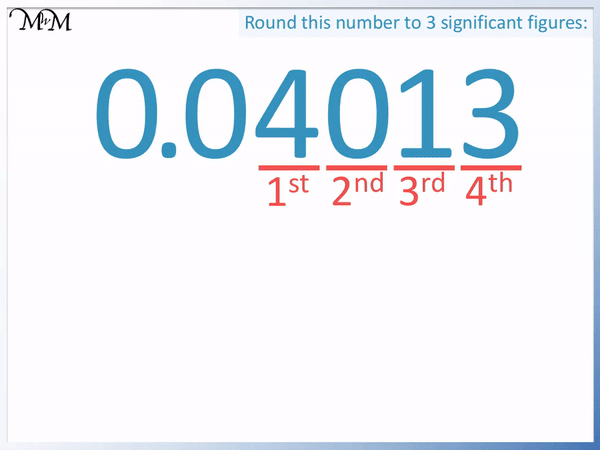• We draw a line after the significant figure that we are rounding to.
• We are rounding to 3 significant figures so we draw a line after the 3rd significant figure of 1.
• We look at the digit after the line to decide whether to round up or down.
• If it is 5 or more, we round up and if it is 4 or less we round down.
• 3 is 4 or less and so we round down.
• This means that we leave the 1 before the line as a 1.
• The digit after the line becomes a zero.
• We do not write zeros on the end of decimals and so, we remove it.
• 0.04013 rounded to 3 significant figures is 0.0401.

Count significant figures from the first non-zero digit.

If the next digit is 5 or more, round up or if it is 4 or less, round down.• We count significant figures from the first digit that is not zero.
• The first digit is 5, which is not zero and so, we start counting.
• 5 is the first significant figure, 4 is the 2nd and 9 is the 3rd.
• 549 has 3 significant figures.• We are rounding 549 to 2 significant figures, so we draw a line after the second significant figure of 4.
• We look at the number after the line to decide whether to round up or down.
• If this number is 5 or more, we round up and if it is 4 or less, we round down.
• 9 is 5 or more and so we round up.
• To round up we increase the number before the line by 1 and change the number after the line to a 0.
• 549 rounds up to 550 when written to 2 significant figures.# How to Round a Number to Significant Figures

## Rules for Rounding Off Significant Figures

Rounding means to simplify a number by writing it to a number that it is close to.

To round a number off to significant figures use these steps:

1. Read the digits of the number from left to right.
2. Start counting the digits from the first digit that is not zero.
3. Count the digits until you get to the significant figures required.
4. Draw a line after this number.
5. If the number after the line is 5 or more, round up or if it is 4 or less, round down.
6. To round up, add 1 to the number before the line and change the numbers after the line to 0.
7. To round down, keep the number before the line the same and change the numbers after the line to 0.

We will look at some examples of rounding numbers to significant figures.

## Rounding a Whole Number to Significant Figures

To round a whole number to a given significant figure, look at the digit after the significant figure required. If this digit is 5 or more, round up or if it is 4 or less, round down.

To round a whole number up, increase the significant figure required by 1 and change the digits that follow it to zero.

To round a whole number down, keep the significant figure required as it is and change the digits that follow it to zero.

In this example, we have 549.

Reading from left to right, the first digit is 5, which is not 0. So we start counting.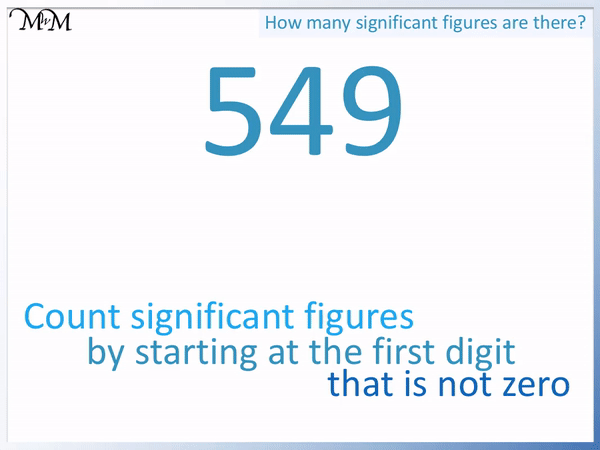5 is the first significant figure.

4 is the second significant figure.

9 is the third significant figure.

549 has 3 significant figures.

We will now round 549 to 2 significant figures.

Since we are rounding to 2 significant figures, we draw a line after the 2nd significant figure.

We draw a line after the 4 and look at the number after this line to decide whether to round up or down.If the number is 5 or more, we round up or if the number is 4 or less, we round down.

9 is 5 or more and so, we round up.

To round up, we increase the number before the line by 1 and change the numbers after the line to zeros.

4 becomes 5 and the 9 becomes a zero.

549 rounds up to 550 when rounded to 2 significant figures.

The 2nd significant figure of this number is in the tens column and so we are deciding between rounding to 540 or 550.

Rounding means to write down the number that is closest.

We can see that 549 is one away from 550 on the number line below but 9 away from 540.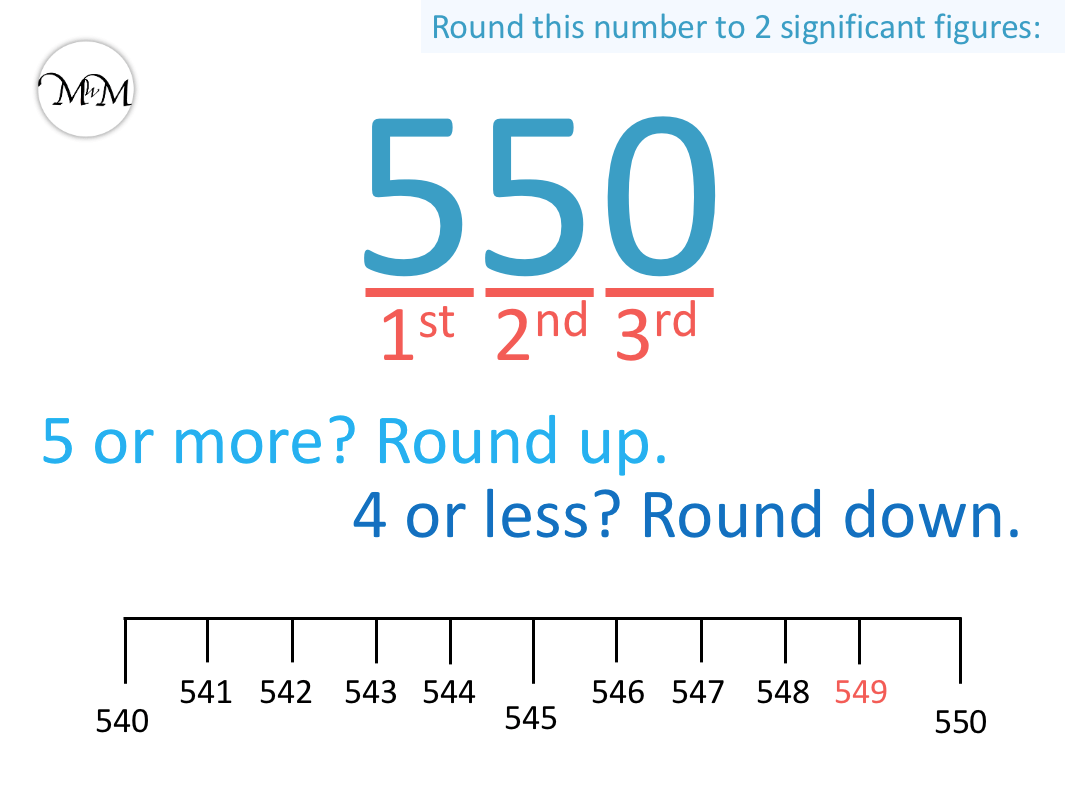549 is nearer to 550 than it is to 540.

We will now round 549 to 1 significant figure.

The first significant digit is the 5 in the hundreds column. This means we have a choice of rounding 549 to 500 or to 600.

The first significant figure in 549 is the 5. We draw the line after this digit.

We look at the digit after the line to decide whether to round up or down.The digit after the line is a 4. It is 4 or less and so we round down.

To round 549 down, we keep the number before the line the same and change the numbers after the line into zeros.

549 rounds down to 500 when written to 1 significant figure.

This means that 549 is nearer to 500 than it is to 600.

Because all whole numbers begin with a non-zero digit, a whole number has the same number of significant figures as it has digits. All of its digits are significant.

Simply count the digits in a whole number to see how many significant digits it has.

## Rounding Decimal Numbers to Significant Figures

To round a decimal to a given number of significant figures, look at the digit after the significant figure required. If it is 5 or more, the number rounds up or if it is 4 or less, the number rounds down.

To round a decimal up, the significant figure increases by 1 and the rest of the digits that follow this digit are removed.

To round a decimal down, the significant figure remains the same and the rest of the digits that follow this digit are removed.

When rounding decimals to significant figures it is important to remember that zeros at the beginning of the number are not significant digits.

We only start counting significant figures from the first digit that is not zero.

In this first example we have 0.0471.

This number has two zero digits at the front, which we do not count.The 4 is the first digit that is not zero and so, we start counting at 4.

4 is the first significant figure.

7 is the second significant figure.

1 is the third significant figure.

We will now round 0.0471 to 1 significant figures.

The first significant figure is the 4 in the hundredths column. We have the choice of keeping the 4 as 4 or rounding it up to a 5.

The choice is to round down to 0.04 or round up to 0.05.

We draw a line after the 4 and look at the next digit after the line to decide how to round off this number.The next digit is a 7 and it is 5 or more. We round up.

To round up, the 4 becomes a 5 and the digits after the line become zero.

0.0471 is nearer to 0.0500 than it is to 0.04,

0.0471 rounds up to 0.0500. However we do not write zeros at the end of a decimal number and instead we write 0.0500 as 0.05.

0.0471 rounds up to 0.05.

In this next example of rounding a decimal to significant figures we have 0.25.

Remember that we do not start counting the digits until we have a digit that is not zero.

We ignore the 0 at the start of 0.25 and start counting at the 2.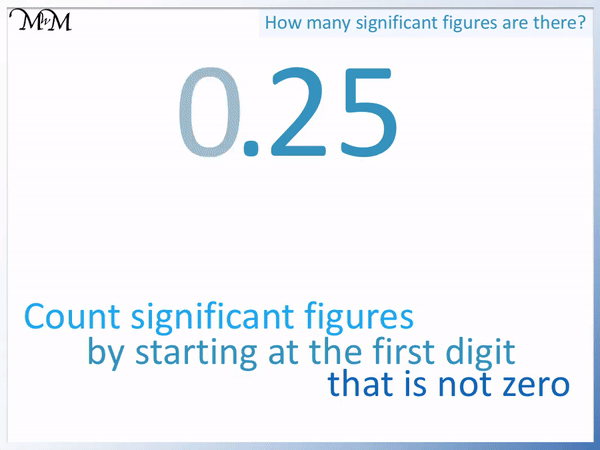2 is the first significant figure.

5 is the second significant figure.

We will now round 0.25 to 1 significant figure.

2 is our first significant figure and so we draw our line after it.We look at the next digit along to decide whether to round up or down.

The next digit is a 5.

5 is included in ‘5 or more’ and so we round up.

We increase the 2 to a 3 and the digits after the line are changed to 0.

0.25 rounds up to 0.30. However we do not write zeros at the end of a decimal number. We write 0.30 as 0.3.

We say that 0.25 rounds up to 0.3.

0.25 is exactly half way between 0.2 and 0.3. We choose to round up numbers that end in the digit 5.

This is because if any other digit came after the 5, the number would round up.

In this example, 0.259 would round up, 0.251 would round up and even 0.250001 would round up. All of these numbers are nearer to 0.3 than 0.2. We include 0.25 so that we have a consistent rule for rounding.

In this next example we have 0.04013.

We have 2 zeros at the beginning of this decimal number and so we do not count these as significant figures.

The first non-zero digit is the 4.4 is the first significant figure.

0 is the second significant figure. We count this zero because we have started counting the significant figures with 4. This 0 comes after a non-zero digit so it is counted.

1 is the third significant figure.

3 is the fourth significant figure.

We will now round the decimal 0.04013 to 3 significant figures.

1 is our third significant figure and so we draw our line after the 1.

We look at the next digit along, after the line, to decide whether to round up or down.The digit after the line is a 3. It is 4 or less and so we round down.

The 1 remains as a 1 and the digits after the line are removed.

The decimal number 0.04013 is rounded down to 0.0401 when written to 3 significant figures.

## Examples of Rounding to Significant Figures

This table contains some examples of rounding different numbers to 1, 2 or 3 significant figures.

Number 1 Significant Figure 2 Significant Figures 3 Significant Figures
8158 8000 8200 8160
6.711 7 6.7 6.71
0.67351 0.7 0.67 0.674
0.03094 0.03 0.031 0.0309
2.103411 2 2.1 2.10

8158 is rounded down to 8000 when written to 1 significant figure. This is because the 1 is ‘4 or less’.

8158 is rounded up to 8200 when written to 2 significant figures. This is because the 5 is ‘5 or more’ and rounds 1 up to 2.

8158 is rounded up to 8160 when written to 3 significant figures. This is because 8 is ‘5 or more’ and rounds the 5 up to a 6.

6.711 rounds up to 7 when written to 1 significant figure. This is because the 7 is ‘5 or more’ and rounds 6 up to 7.

6.711 rounds down to 6.7 when written to 2 significant figures. This is because the first 1 is ‘4 or less’.

6.711 rounds down to 6.71 when written to 3 significant figures because the second 1 is ‘4 or less’.

0.67351 rounds up to 0.7 when written to 1 significant figure because the 7 is ‘5 or more’ and rounds the 6 up to a 7.

0.67351 rounds down to 0.67 when written to 2 significant figures because the 3 is ‘4 or less’.

0.67351 rounds up to 0.674 when written to 3 significant figures because the 5 is ‘5 or more’ and rounds the 3 up to a 4.

0.03094 rounds down to 0.03 when written to 1 significant figure because the 0 after the 3 is ‘4 or less’.

0.03094 rounds up to 0.031 when written to 2 significant figures because the 9 is ‘5 or more’ and rounds the 0 up to a 1.

0.03094 rounds down to 0.0309 when written to 3 significant figures because the 4 is ‘4 or less’.

2.103411 rounds down to 2 when written to 1 significant figure because the 1 is ‘4 or less’.

2.103411 rounds down to 2.1 when written to 2 significant figures because the 0 is ‘4 or less’.

2.103411 rounds down to 2.10 when written to 3 significant figures because the 3 is ‘4 or less’. We can write this number as 2.1 or 2.10 but we will write 2.10 because the question asks for 3 significant figures.Now try our lesson on Converting Decimals to Fractions where we learn how to write decimals as fractions.error: Content is protected !!